# Basic Geometrical Ideas Class 6 Worksheet pdf

Please check Solutions of Basic Geometrical Ideas Class 6 Worksheet pdf at the end of the questions.Q.1) _______ has no end point.
[A] Line segment
[B] Line
[C] Ray

Q.2) _______ has two end points.
[A] Line segment
[B] Line
[C] Ray

Q.3) _______ has only one end point.
[A] Line segment
[B] Line
[C] Ray

Q.4) Which of the following does have a definite length?
[A] Line segment
[B] Line
[C] Ray

Q.5) _____ lines can pass through a single point.
[A] Only one
[B] two
[C] Infinite

Q.6) _____ line can pass through two given points.
[A] Only one
[B] Infinite

Q.7) A ray has _____ length.
[A] indefinite
[B] definite

Q.8) A line has _____ length.
[A] indefinite
[B] definite

Q.9) A line segment has _____ length.
[A] indefinite
[B] definite

Q.10) ______ can be drawn on a paper.
[A] Line segment
[B] Ray
[C] Line

Q.11) An angle which has a measure of 50˚ is ?
[A] an obtuse angle
[B] an acute angle
[C] a right angle

Q.12) An angle which has a measure of 90˚ is ?
[A] a straight angle
[B] a reflex angle
[C] a right angle

Q.13) 4 right angles = ?
[A] 540˚
[B] 450˚
[C] 360˚

Q.14) 5/2 right angles = ?
[A] 225˚
[B] 335˚
[C] 360˚

Q.15) Which of the following figure is a polygon?

[A]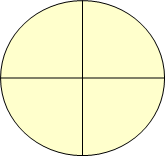[B]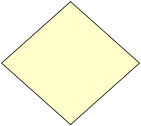[C]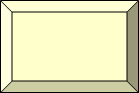Q.16) ______ is a simple closed figure formed by 3 or more than 3 sides.
[A] Polygon
[B] Circle
[C] Line segment

Q.17) Triangle has ______ sides.
[A] 3
[B] 4
[C] 2

Q.18) Pentagon has ______ vertices.
[A] 4
[B] 3
[C] 5

[A] 3
[B] 4
[C] 5

Q.20) Pentagon has ______ sides.
[A] 4
[B] 3
[C] 5

[A] 3
[B] 4
[C] 5

Q.22) A polygon having 3 sides is called a ______ .
[A] triangle
[B] hexagon

Q.23) Which of the following figure is a closed figure?

[A]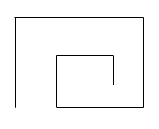[B]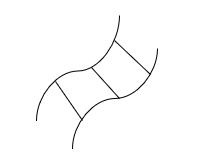[C]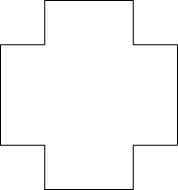Q.24) Which of the following figure is an open figure?

[A]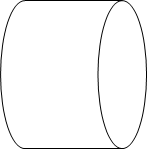[B]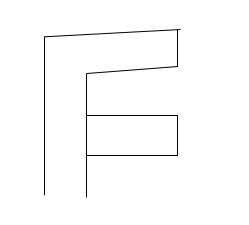[C]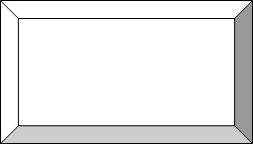Q.25) State whether the given statement is true or false for the given figure:
AF is parallel to BC.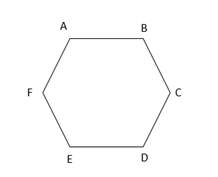[A] True
[B] False

Q.26) A line segment which passes through the center of a circle , and whose end points lie on the circumference of the circle is called the  ______ of a circle.
[A] diameter
[B] secant
[C] arc

Q.27) Chord that divide the circle into two equal parts is called _______ of the circle.
[A] diameter
[B] sector
[C] secant

Q.28) If the radius of the circle is 7 cm, find its diameter?
[A] 14 cm
[B] 21 cm
[C] 28 cm

Q.29) If the diameter of the circle is 12 cm, find its radius?
[A] 24 cm
[B] 6 cm
[C] 18 cm

Q.30) Is the given figure is symmetrical about the dotted line?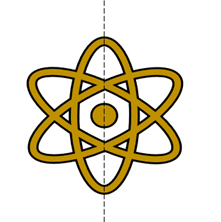[A] Yes
[B] No

Q.31) Given below is a bar graph showing the number of students passed in different subjects.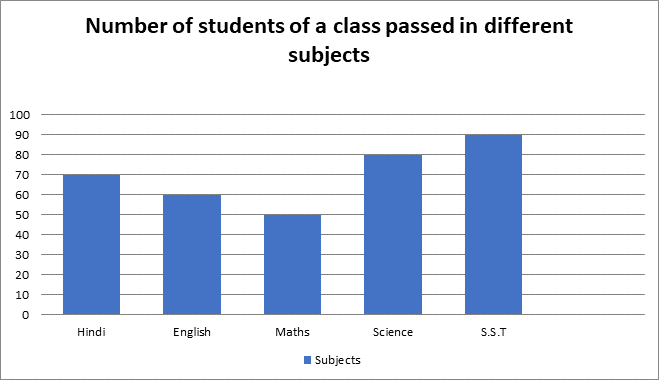In which subject Maximum number of students have passed ?
[A] Hindi
[B] Science
[C] S.S.T

### Basic Geometrical Ideas Class 6 Worksheet pdf – Explanations

Q.1) Explanation:

Line has no end point.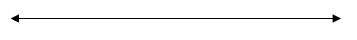Q.2) Explanation:

Line segment has two end points.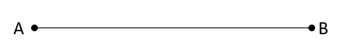In the given diagram, A and B are the two end points.

Q.3) Explanation:

Ray has only one end point.

In the given diagram, A is the only end point. The Ray goes indefinitely in the other direction till infinity.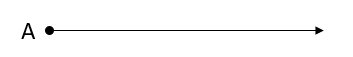Q.4) Explanation:

Line segment has a definite length.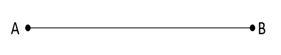In the given diagram, A and B are the two end points of Line segment AB.

In case of Line, there are no end points and therefore it has no definite length.In the case of Ray, A is the only end point. The Ray goes indefinitely in the other direction till infinity, and therefore it has no definite lengthQ.5) Explanation:

Infinite lines can pass through a single point.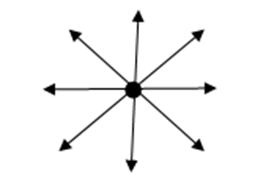Q.6) Explanation:

Only one line can pass through two given points. If A and B are two points, only one line can pass between these two Points.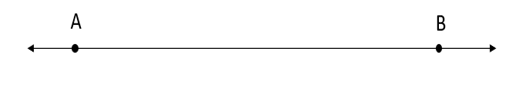Q.7) Explanation:

A ray is a part of a line starting from one point and it can be extended infinitely.

Hence, a ray has indefinite length.Q.8) Explanation:

A line can be extended from both the sides infinitely.

Hence, a line has indefinite length.Q.9) Explanation:

Both the sides of line segment has end points, so it can’t be extended from any of its ends.

Hence, a line segment has definite length.Q.10) Explanation:

A Line segment can be drawn on a paper, since it has definite length.

However, a ray or line can’ t be draw on paper as they have indefinite length.Q.11) Explanation:

Acute angle – If the measure of an angle is more than 0˚ but less than 90˚ , it is called an acute angle.
Obtuse angle – If the measure of an angle is more than 90˚ but less than 180˚ , it is called an obtuse angle.
Right angle – If the measure of an angle is 90˚, then the angle is called a right angle.
In present case,
The given angle is 50˚
We know that
0˚ < 50˚ < 90˚
Hence, an angle which has a measure of 50˚ is an acute angle.

Q.12) Explanation:

Straight angle – If the measure of an angle is 180˚, then the angle is called a straight angle.
Reflex angle – If the measure of the angle is more than 180˚ but less than 360˚ , then the angle is called a reflex angle.
Right angle – If the measure of an angle is 90˚, then the angle is called a right angle.
In present case,
Measure of the given Angle is = 90˚
Hence, an angle which has a measure of 90˚ is a right angle.

Q.13) Explanation:

We know that,
If the measure of an angle is 90˚, then the angle is called a right angle.
In other words,
1 right angle = 90˚
4 right angles = 4 x 90˚
4 right angles = 360˚

Q.14) Explanation:

As we know that,
If the measure of an angle is 90˚, then the angle is called a right angle.
In other words,
1 right angle = 90˚
5/2 right angles = 5/2 x 90˚
5/2 right angles = 225˚

Q.15) Explanation:

Polygon is : –
·  A simple closed figure
·  Having three or more than three sides
From the given figures, the Polygon isOption 1 and 3 have more than 3 sides but they are not simple closed figures as they intersect themselves at some point.

Q.16) Explanation:

Polygon is a simple closed figure formed by 3 or more than 3 sides.

Q.17) Explanation:

Triangle has 3 sides.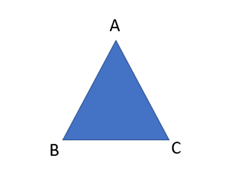In the given diagram, the 3 sides Triangle are AB, BC and AC.

Q.18) Explanation:

Pentagon has 5 vertices.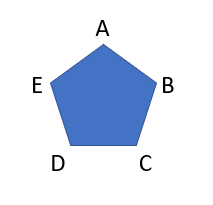In the given figure, the vertices of the Pentagon are marked as A, B, C, D and E.

Q.19) Explanation: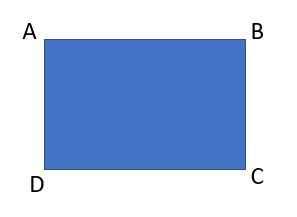In the given diagram, the 4 sides of Quadrilateral are AB, BC, CD and AD.

Q.20) Explanation:

Pentagon has 5 sides.In the given diagram, the 5 sides of Pentagon are AB, BC, CD, DE and AE.

Q.21) Explanation: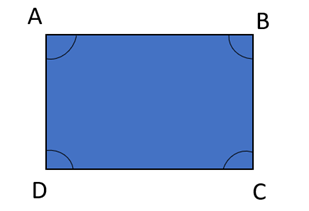In the given figure, the angles of the Quadrilateral are marked as ∠A, ∠B, ∠C, ∠D.

Q.22) Explanation:

A polygon having 3 sides is called a triangle.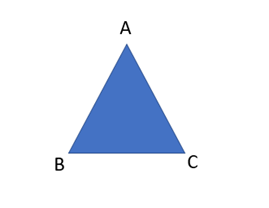In the given diagram, the 3 sides of the given figure are AB, BC and AC.

Q.23) Explanation:

If starting point and the end point of a figure are same, then the figure is a closed figure.

So, the closed figure amongst the given figures isQ.24) Explanation:

If starting point and the end point of a figure are not same, then the figure is an open figure.

So, the open figure amongst the given figures isQ.25) Explanation:

When two lines are equidistant from each other and can never meet at any point, they are called parallel lines.In given figure, we observe that
Line AF and Line BC are not equidistant from each other
Hence, we can say that Line AF is not parallel to Line BC.

Q.26) Explanation:

A line segment which passes through the center of a circle , and whose end points lie on the circumference of the circle is called the Diameter of a circle.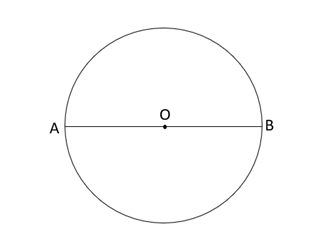In the above figure, AB, is a line segment which passes through the center O , and its end points (A and B) lie on the circumference of the circle.
Hence, AB is the diameter of the given Circle.

Q.27) Explanation:

Chord that divides the circle into two equal parts is called Diameter of the circle.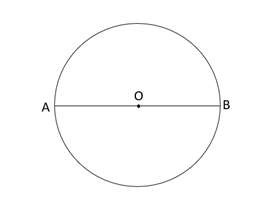Here, AB is the chord , which is the diameter of a circle, as it divides the circle into two equal parts.

Q.28) Explanation:

As we know that,
Diameter of a Circle = 2 x ( Radius of a Circle )
Given,
then,
Diameter of a Circle = 2 x 7 cm
Diameter of a Circle = 14 cm
Hence, the Diameter of a Circle is 14 cm

Q.29) Explanation:

Given,
Diameter of a Circle = 12 cm
We know that,
Diameter of a Circle = 2 x ( Radius of a Circle )
or in other words
Radius of a Circle = Diameter of a Circle/2
Radius of a Circle = 12/2 cm
Radius of a Circle = 6 cm
Hence, the Radius of a Circle is 6 cm

Q.30) Explanation:

A figure is said to be symmetrical about the dotted line, if on folding, one part will fit exactly over the other part.
If we separate the given image on the dotted line, it would be like this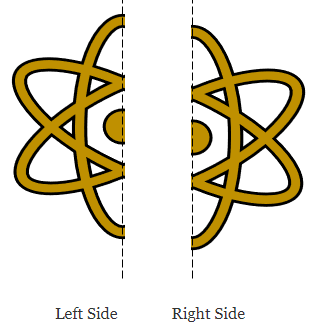Since the image on the left side of the dotted line is same in shape from the image on the right side of the dotted line, although its different in appearance, on folding left side image would exactly fit over the right side image. Hence, we can say that the given figure is symmetrical about dotted line.

Q.31) Explanation:

We have to find the subject in which maximum number of students have passed. The bar graph which has the tallest length would represent the subject, in which maximum number of students have passed

Look at the bar graph which is the tallest one amongst the given graphs.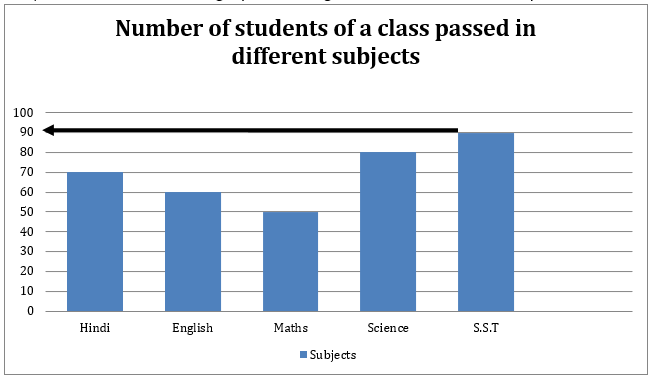According to the bar graph S.S.T is the tallest bar. Therefore, the maximum number of students have passed in SST.